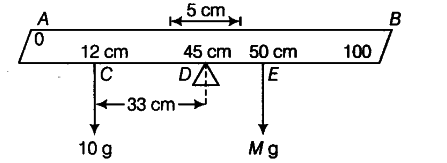# A metre stick is balanced on a knife edge at its centre

A metre stick is balanced on a knife edge at its
centre. When two coins, each of mass 5 g are put one on top of the other at the 12 cm mark, the stick is found to be balanced at 45.0 cm. What is the mass of the metre stick.

Let total mass of the metre stick be M kg.Distance between mid-point E and new centre of gravity (DA),
= 50 — 45 = 5 cm
Distance between 12 cm mark and new centre of gravity (CD),
= 45-12 = 33 cm
From principle of moments in equilibrium,
M x DE = (2 x 5) x CD Mx 5 = 10x33 or M =66 g
.’… Mass of the metre stick is 66 g